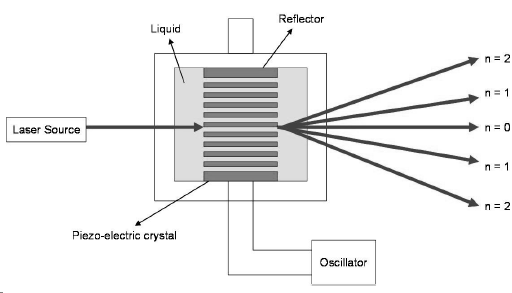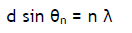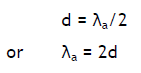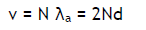Acousing Grating: Principle: When ultrasonic waves are passed through a liquid, the density of the liquid varies layer by layer due to the variation in pressure and hence the liquid will act as a diffraction grating, so called acoustic grating. Under this condition, when a monochromatic source of light is passed through the acoustical grating, the light gets diffracted. Then, by using the condition for diffraction, the velocity of ultrasonic waves can be determined.Construction & Working: The liquid is taken in a glass cell. The Piezo-electric crystal is fixed at one side of the wall inside the cell and ultrasonic waves are generated. The waves travelling from the crystal get reflected by the reflector placed at the opposite wall. The reflected waves get superimposed with the incident waves producing longitudinal standing wave pattern called acoustic grating. If light from a laser source such as He-Ne or diode laser is allowed to pass through the liquid in a direction perpendicular to the grating, diffraction takes place and one can observe the higher order diffraction patterns on the screen. The angle between the direct ray and the diffracted rays of different orders (θn) can be calculated easily. According to the theory of diffraction,....1

where n = 0, 1, 2, 3, … is the order of diffraction, B is the wavelength of light used and d is the distance between two adjacent nodal or anti-nodal planes.
Knowing n, θn and B, the value of d can be calculated from eqn. (1). If λa is the wavelength of the ultrasonic waves through the medium, then...............2

If the resonant frequency of the Piezo-electric oscillator is N, then the velocity of ultrasonic wave is given by.....................3

This method is useful in measuring the velocity of ultrasonic waves through liquids and gases at various temperatures. From these measurements, many parameters of the liquid such as free volume, compressibility, etc., can be calculated.